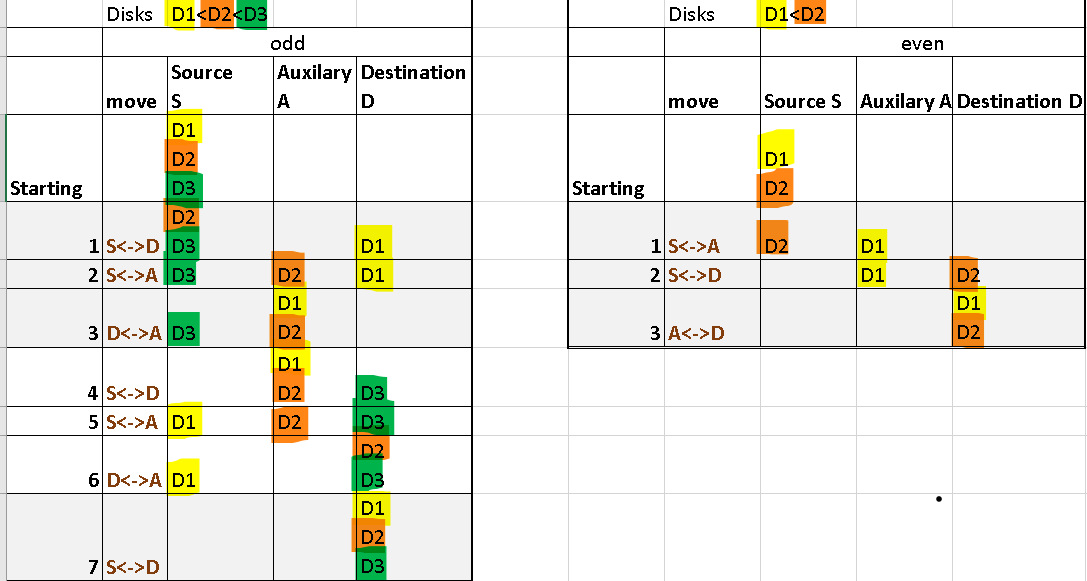# Tower of Hanoi (Recursive and Iterative approach)

The Tower of Hanoi (also called the Tower of Brahma or Lucas’ Tower and sometimes pluralized as Towers) is a mathematical game or puzzle. It consists of three rods and a number of disks of different sizes, which can slide onto any rod.

The puzzle starts with the disks in a neat stack in ascending order of size on one rod, the smallest at the top, thus making a conical shape.

The objective of the puzzle is to move the entire stack to another rod, obeying the following simple rules:

1. Only one disk can be moved at a time.
2. Each move consists of taking the upper disk from one of the stacks and placing it on top of another stack or on an empty rod.
3. No larger disk may be placed on top of a smaller disk.

With 3 disks, the puzzle can be solved in 7 moves. The minimal number of moves required to solve a Tower of Hanoi puzzle is 2n − 1, where n is the number of disks.

# Iterative approach

Source S, Destination D, Auxilary A

Iterative pseudocode

if disks are odd or even
if even
for (2 PW n)-1 times follow below these three steps
1. move S disk to A or vice versa depending upon bigger of the two (i%3==1)
2. move S disk to D or vice versa depending upon bigger of the two (i%3==2)
3. move A disk to D or vice versa depending upon bigger of the two (i%3==0)
Repeat above steps till loop is complete
if odd
for (2 PW n)-1 times follow below these three steps
1. move S disk to D or vice versa depending upon bigger of the two (i%3==1)
2. move S disk to A or vice versa depending upon bigger of the two (i%3==2)
3. move D disk to A or vice versa depending upon bigger of the two (i%3==0)
Repeat above steps till loop is completeDry Run when disks are odd or even in number

Refining further

if disk are odd or even (n%2 == 0)
temp = D
D = A
A = temp
//Basically interchanging Destination and Auxilary

for (2 PW n)-1 times follow below these three steps
1. move S disk to D or vice versa depending upon bigger of the two (i%3==1)
2. move S disk to A or vice versa depending upon bigger of the two (i%3==2)
3. move D disk to A or vice versa depending upon bigger of the two (i%3==0)
Repeat above steps till loop is complete

For code refer https://www.geeksforgeeks.org/iterative-tower-of-hanoi/

# Recursive Approach

recursive pseudocode

Base Case: if(n==1) move S disk to D and exit
1. move (n-1) disks from S to A
2. move n th disk from S to D
3. move (n-1) disks from A to D

Code is shown below:

public class Solution {
public static void main(String[] args) {
Scanner sc = new Scanner(System.in);
int rings = sc.nextInt();
solve(rings, “A”, “B”, “C”);
}

static void solve(int rings, String source, String target, String helper){
if(rings>0){
//Move n-1 top rings to helper peg
solve(rings-1, source, helper, target);
//Move nth ring to target peg
System.out.println(“Moving ring “+rings+” from “+source+” to “+target);
//Move n-1 top rings from helper to target;
solve(rings-1, helper, target, source);
}
}
}

# Variations

DOUBLE DECKER In this variation, called Double Decker, we duplicate every disk to create a stack of 2n disks with two of each size as shown in Figure.

For the convenience of notation, we will consider (only for this variant) that a stack of height n has 2n disks. Fig. Double Decker for n = 3. A trivial solution to Double Decker is to simply treat it as a standard instance of the Tower of Hanoi with 2n disks and, thus, will need the usual 2 2n − 1 = 4n − 1 move. This trivial solution, however, does not benefit from equal-size disks. For instance, if we do not require that disks of the same size must preserve their original order, then a better solution for Double Decker is to emulate the standard Tower of Hanoi solution by duplicate moves, to give a0 = 0, a1 = 2, a2 = 6, …

The algorithm is shown below.

DoubleDecker(n, x, y, z)
-if n > 0
— -then DoubleDecker(n − 1, x, z, y)
— — — Move(1, x, z)
— — — Move(1, x, z)
— — — DoubleDecker(n − 1, y, x, z)

References:

--

--